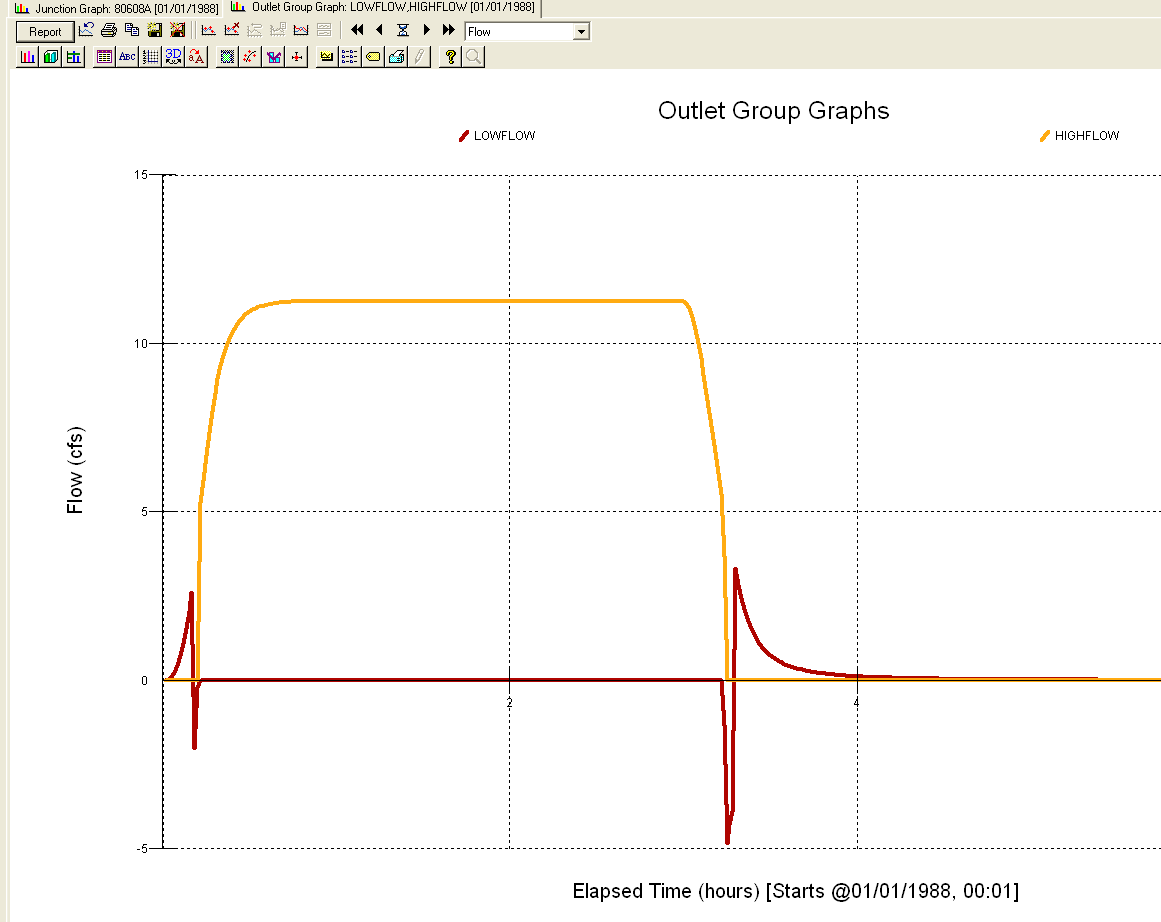#INFOSWMM

# How to Divide the Inflow at a Node in InfoSWMM

Subject:  How to Divide the Inflow at a Node in InfoSWMM

In SWMM 5 only the Kinematic Wave solution allows a flow divider at a node to divide the Inflow to node to two  downstream  links, but you can use the Inflow/Outflow Outlet type in InfoSWMM to divide the inflow based on a Inflow/Outflow Diversion Table (Figure 1).  For example, in InfoSWMM it is possible to have two downstream links from a Node that are Outlet types Inflow/Outflow so that the low flow goes down one link and the high flow goes down the other link (Figure 2 and Figure 3).   The low flow and the high flow  link  use different diversion tables in which the tables are constructed so that the flow is positive in one link and zero in the other to a dividing flow value and then zero and positive for the same two links after the dividing flow value ( 5 cfs in the example).

Figure 1.  Types of OUTLETS in InfoSWMM and SWMM 5Figure 2.  Example low flow and high flow Outlet Links to divide the total  inflow at the upstream node at 5 cfs.Figure 3.   The flow is divided into the low and high flow links at the dividing flow of 5 cfs.